# 【Unity C#编程】图表 可视化数据：创建图表 – 9TECH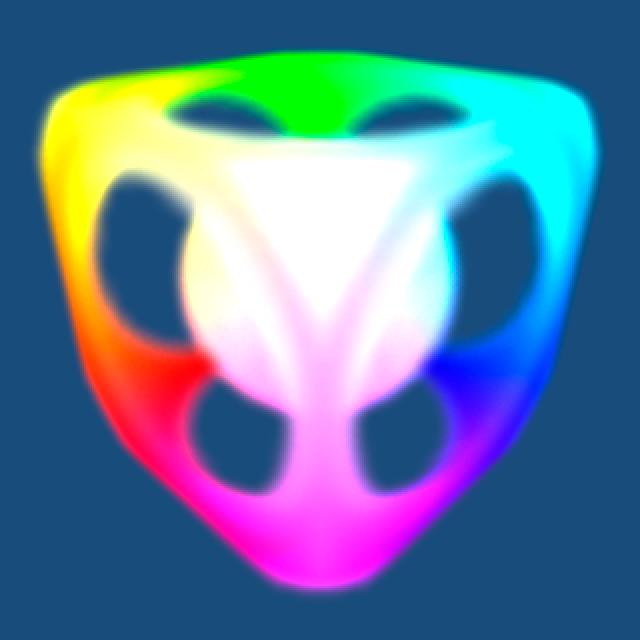• 创建图表，从简单到复杂
• 控制粒子系统
• 写各种数学函数
• play模式下改变行为
• 使用Unity事件函数Start Update
• 写循环，包括单循环和嵌套循环
• 使用数组、枚举和代表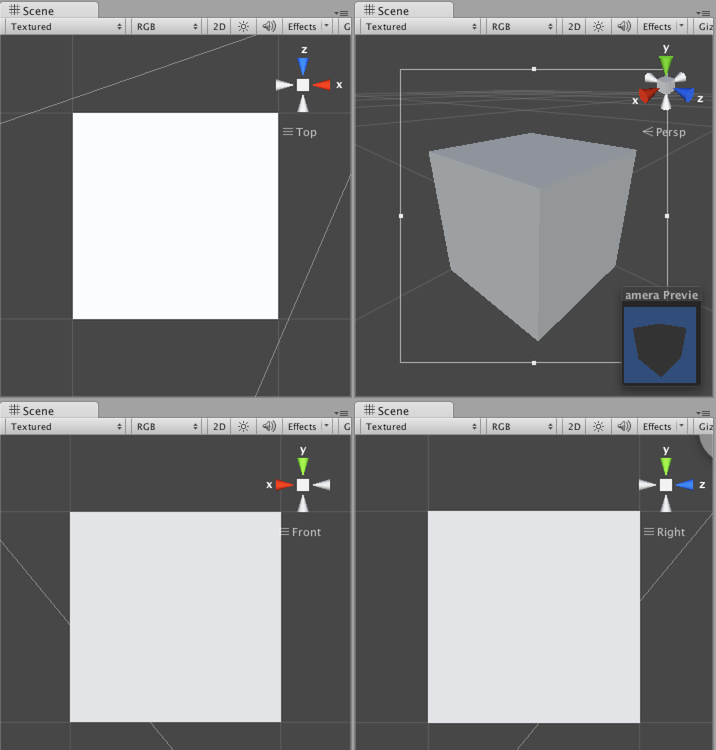```using UnityEngine;
public class Grapher1 : MonoBehaviour {}```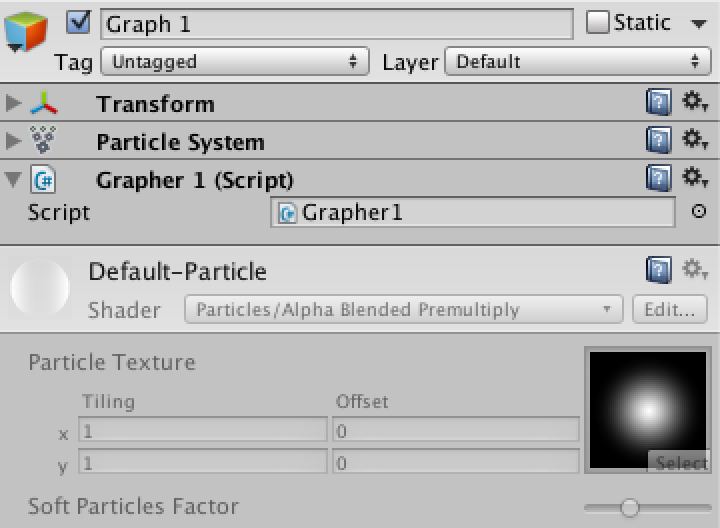```using UnityEngine;
public class Grapher1 : MonoBehaviour {
public int resolution = 10;
private ParticleSystem.Particle[] points;
void Start () {
points = new ParticleSystem.Particle[resolution];
}
}```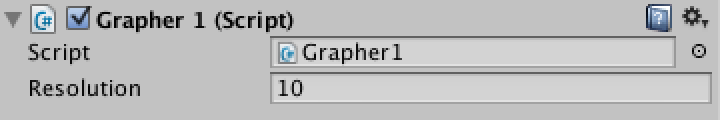Grapher1配置分辨率

```void Start () {
if (resolution < 10 || resolution > 100) {
Debug.LogWarning("Grapher resolution out of bounds, resetting to minimum.", this);
resolution = 10;
}
points = new ParticleSystem.Particle[resolution];
}```

现在该把点沿着X轴放置。第一个点应该放在0，最后一个放在1。其他的点应该在这两者之间。所以距离，或者说X增量，两点之间是1（分辨率-1）。

```void Start () {
if (resolution < 10 || resolution > 100) {
Debug.LogWarning("Grapher resolution out of bounds, resetting to minimum.", this);
resolution = 10;
}
points = new ParticleSystem.Particle[resolution];
float increment = 1f / (resolution - 1);
for (int i = 0; i < resolution; i++) {
float x = i * increment;
points[i].position = new Vector3(x, 0f, 0f);
points[i].color = new Color(x, 0f, 0f);
points[i].size = 0.1f;
}
}```

```void Update () {
particleSystem.SetParticles(points, points.Length);
}```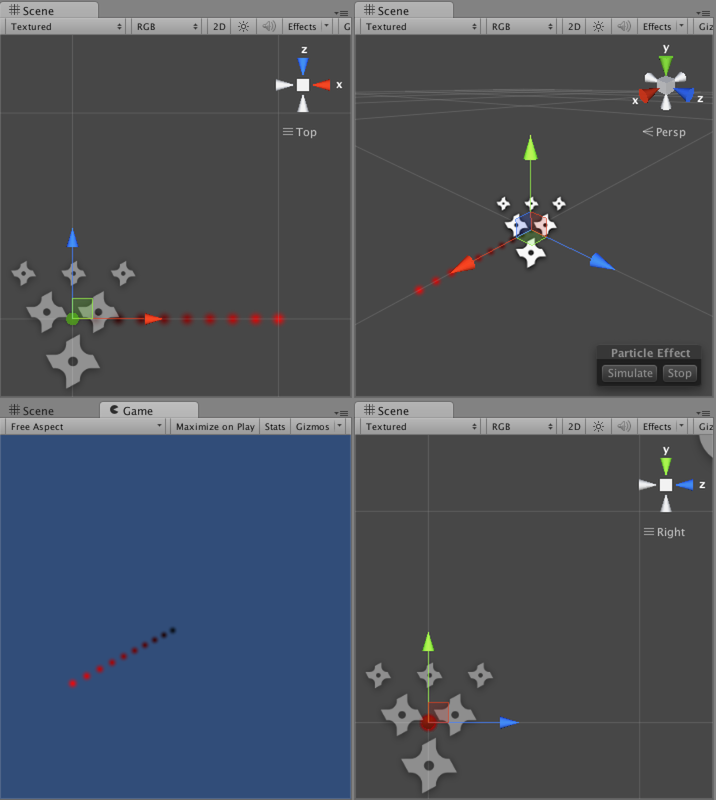10粒子组成的线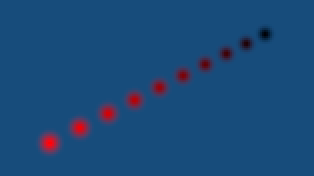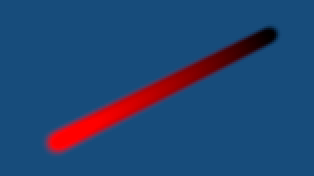```using UnityEngine;

public class Grapher1 : MonoBehaviour {

public int resolution = 10;

private int currentResolution;
private ParticleSystem.Particle[] points;

void Start () {
CreatePoints();
}

private void CreatePoints () {
if (resolution < 10 || resolution > 100) {
Debug.LogWarning("Grapher resolution out of bounds, resetting to minimum.", this);
resolution = 10;
}
currentResolution = resolution;
points = new ParticleSystem.Particle[resolution];
float increment = 1f / (resolution - 1);
for(int i = 0; i < resolution; i++){
float x = i * increment;
points[i].position = new Vector3(x, 0f, 0f);
points[i].color = new Color(x, 0f, 0f);
points[i].size = 0.1f;
}
}

void Update () {
if (currentResolution != resolution) {
CreatePoints();
}
particleSystem.SetParticles(points, points.Length);
}
}```

现在只要改变分辨率的值就能重建图表了。然而，你会注意到每当分辨率超出范围，甚至是输入的时候，控制台都会弹出警告。我们可以使用Range属性来告诉Unity编辑器使用滑块来代替数字框。

```[Range(10, 100)]
public int resolution = 10;``````void Update () {
if (currentResolution != resolution) {
CreatePoints();
}
for (int i = 0; i < resolution; i++) {
Vector3 p = points[i].position;
p.y = p.x;
points[i].position = p;
}
particleSystem.SetParticles(points, points.Length);
}``````void Update () {
if (currentResolution != resolution) {
CreatePoints();
}
for (int i = 0; i < resolution; i++) {
Vector3 p = points[i].position;
p.y = p.x;
points[i].position = p;
Color c = points[i].color;
c.g = p.y;
points[i].color = c;
}
particleSystem.SetParticles(points, points.Length);
}``````using UnityEngine;

public class Grapher1 : MonoBehaviour {

[Range(10, 100)]
public int resolution = 10;

private int currentResolution;
private ParticleSystem.Particle[] points;

private void CreatePoints () {
currentResolution = resolution;
points = new ParticleSystem.Particle[resolution];
float increment = 1f / (resolution - 1);
for(int i = 0; i < resolution; i++){
float x = i * increment;
points[i].position = new Vector3(x, 0f, 0f);
points[i].color = new Color(x, 0f, 0f);
points[i].size = 0.1f;
}
}

void Update () {
if (currentResolution != resolution || points == null) {
CreatePoints();
}
for (int i = 0; i < resolution; i++) {
Vector3 p = points[i].position;
p.y = p.x;
points[i].position = p;
Color c = points[i].color;
c.g = p.y;
points[i].color = c;
}
particleSystem.SetParticles(points, points.Length);
}
}```